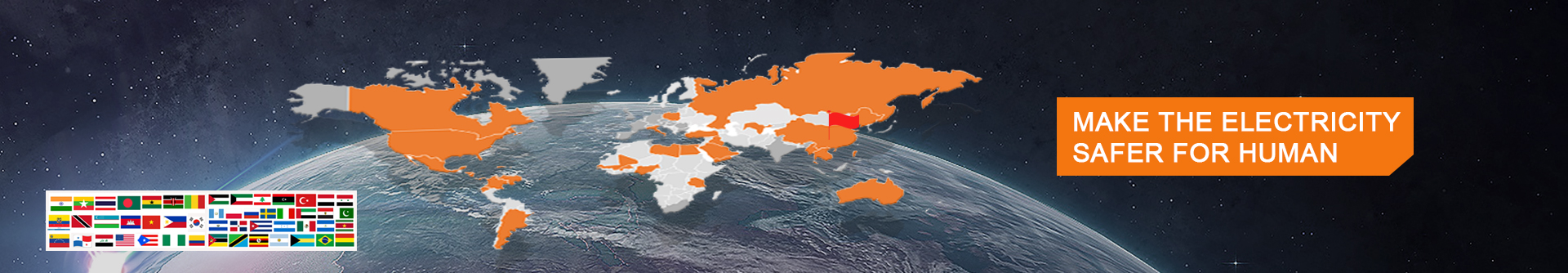# What are power, voltage, current and resistance?

Date：2020-05-16 09:19:31 click：1210

1. Definition of power: power refers to the work done by an object in a unit of time, that is, power is the physical quantity that represents how fast or slow work is done.

The formula for calculating power is P=W/tP, which means power in watts, short for watts, W for work, J for joule, short for coke, J for time, t for seconds, and s for symbol.

P is UI is U2 over R

2. Definition of voltage: electrostatics calls the potential difference between two points voltage.

The cause of the current in a conductor is called voltage.Voltage, like water pressure, which makes still water flow in a certain direction, is a physical quantity that makes electrons in a conductor move in a certain direction.

3. Definition of current: current is the directional movement of charge.

The magnitude of the current is called the current intensity (current for short, symbol I), which refers to the amount of charge passing through A section of the wire per unit time. The amount of charge passing through A coulomb per second is called an "ampere" (A).

The usual units are mA and vigila.

Conversion: 1A=1000mA, and 1mA= 1000a.

4. Resistance is defined as the resistance to the flow of current. The unit of resistance is the ohm, use symbol "Ω said."

Ohm: when a voltage of 1 volt is applied to both ends of a resistor, the resistance of the resistor is 1 ohm if a current of 1 ampere passes through the resistor. In the international system of units, the unit of resistance is the Ω (ohm), in addition to K Ω (M), M Ω (mge). Among them: the unit conversion 1 m Ω Ω = 1000 k, 1 k Ω Ω = 1000.